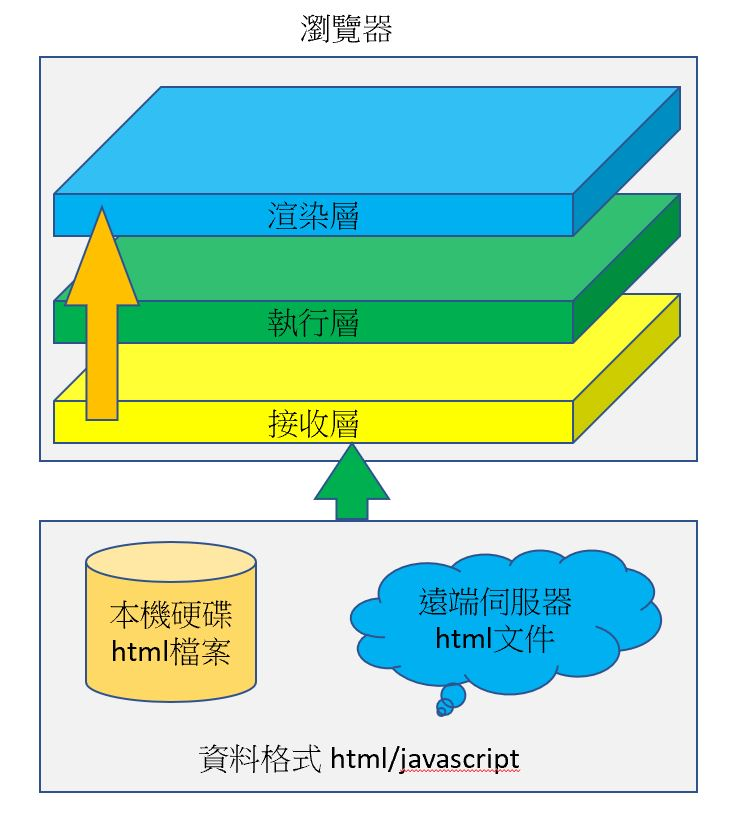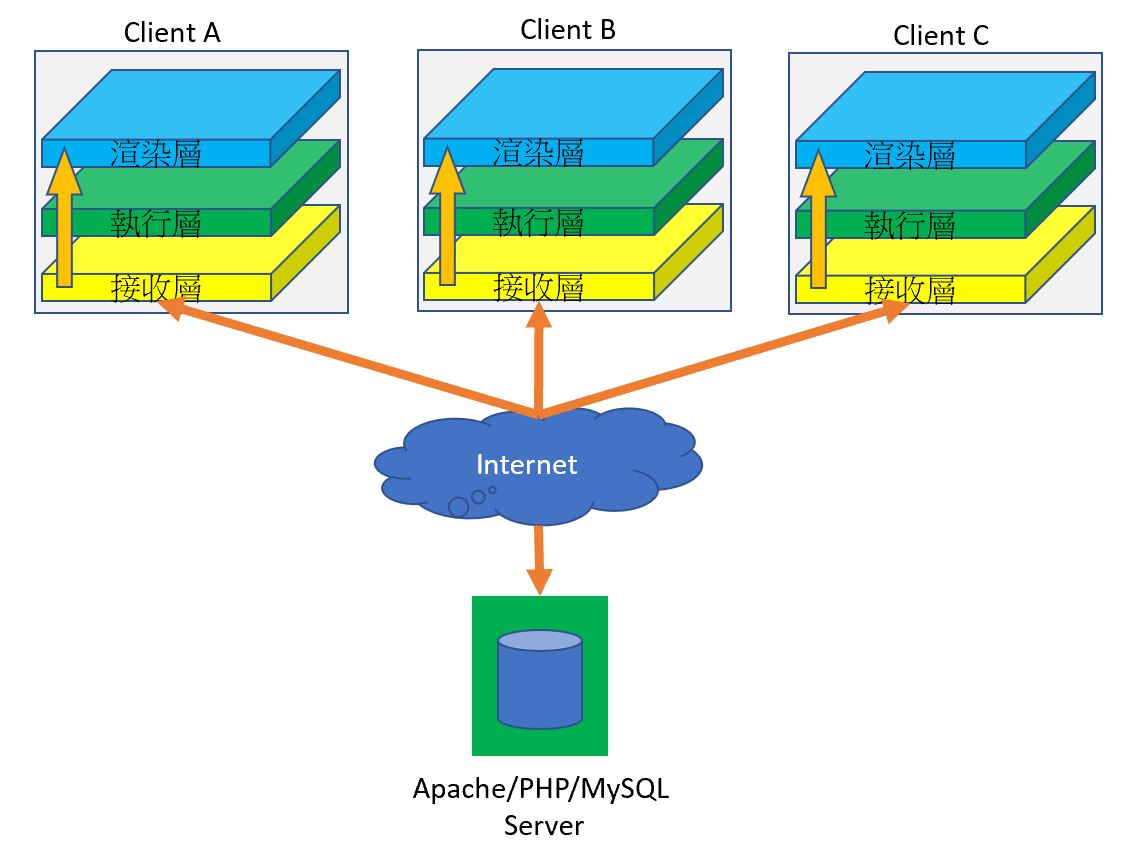# 瀏覽器三層# 網路連線

Server產生的 html文件，會透過Internet傳送到客戶端(Client A,B,C) 的接收層# 後端

<html>   <head>      <title>PHP簡介</title>   </head>   <body>      <table border="1" cellspacing="0" cellpadding="0" align="center">      <?php         for ($i=1;$i<=9;i++){ echo "<tr align='center'>"; for (j=1;$j<=9;$j++){               echo "<td height='20' width='50'>";               echo sprintf("%s*%s=%s", $i,$j, $i*$j);               echo "</td>";            }            echo '</tr>';         }      ?>      </table>   </body></html>

php 字串可以使用單引號 ‘ ‘ 及雙引號 ” “， 這點跟 python 一樣。但php習慣用雙引號，然後把單引號 ‘ ‘ 留給 html及MySQL。請注意，MySQL只能用單引號。

<html>   <head>      <title>JavaScript說明</title>   </head>   <body>      <table border="1" cellspacing="0" cellpadding="0" align="center">      <script language="javascript">         for (i=1;i<=9;i++){            document.write("<tr>");            for (j=1;j<=9;j++){               a=${i}*${j}=${i*j}; document.write("<td height='20' width='50'>"); document.write(a); document.write("</td>"); } document.write("</tr>"); } </script> </table> </body></html> # 如何決擇前後端 通常需要取得伺服器資料庫的工作，就交由後端來執行。絕大部份的伺服器，通常也就只是處理資料庫的資料而以。 至於邏輯演算法，比如 5*3 這種計算式，就交由前端來執行。 不過像 AI 這種演算法，需要高效能的處理圖器才能執行，所以也就只能交由伺服器處理。 # 為何爬不到資料 爬蟲程式的 requests 套件，是直接分析接收層的 html 語法，並不是解析執行層後的資料。為了解決這種問題，只能求助 Selenium，由 Selenium 渲染後，再取得渲染後的 html。 # 前後端應用 此範例使用 php及 form表單新增員工資料庫 資料庫/資料表為 cloud/employee，欄位如下 CREATE TABLE 員工資料表 (id int NOT NULL AUTO_INCREMENT,員工編號 varchar(10) COLLATE utf8_unicode_ci NOT NULL,姓名 varchar(20) COLLATE utf8_unicode_ci NOT NULL,帳號 varchar(10) COLLATE utf8_unicode_ci NOT NULL,密碼 varchar(10) COLLATE utf8_unicode_ci NOT NULL,生日 date DEFAULT NULL,PRIMARY KEY (id)) ENGINE=InnoDB DEFAULT CHARSET=utf8mb3 COLLATE=utf8_unicode_ci 後端 process.php 代碼如下，可以使用如下網址測試 http://localhost/lcc/process.php?userId=0001&userName=小黃&userAccount=kevin&userPassword=1234&userBirthday=1990-05-06 <?php$id=$_GET["userId"];$name=$_GET["userName"];$account=$_GET["userAccount"];$password=$_GET["userPassword"];$birthday=$_GET["userBirthday"]; //echo sprintf("%s, %s, %s, %s, %s",$id, $name,$account, $password,$birthday);
//php從5.0開始，也支援物件導向
$conn=new mysqli("localhost","登入資料庫帳號","登入資料庫密碼","資料庫名稱"); if(!$conn){
die("connect error");
}
$cmd=sprintf( "insert into 員工資料表 (員工編號, 姓名, 帳號, 密碼, 生日) values ('%s', '%s', '%s','%s','%s')",$id, $name,$account, $password,$birthday);
//echo $cmd; if(!$conn->query($cmd)){ die("insert error"); }$conn->close();
echo "insert success";
?>

<!--前端工程師的事, 製作表單填資料--><html>    <head>        <meta charset="UTF-8">        <title>會員註冊系統</title>    </head>    <body>        <form action="process.php" method="get">            <table border="1" cellpadding="0" cellspacing="0" width="250" align="center">                <tr>                    <td width="80">員工編號</td>                    <td>                        <input type="text" name="userId"/>                    </td>                </tr>                <tr>                    <td width="80">姓名</td>                    <td>                        <input type="text" name="userName"/>                    </td>                </tr>                <tr>                    <td width="80">帳號</td>                    <td>                        <input type="text" name="userAccount"/>                    </td>                </tr>                <tr>                    <td width="80">密碼</td>                    <td>                        <input type="password" name="userPassword"/>                    </td>                </tr>                           <tr>                    <td width="80">生日</td>                    <td>                        <input type="text" name="userBirthday"/>                    </td>                </tr>                <tr>                    <td colspan="2" align="center">                        <input type="submit" value="註冊"/>                    </td>                </tr>            </table>        </form>    </body></html>

todo